# The rough graph of the function y = sin x .### Single Variable Calculus: Concepts...

4th Edition
James Stewart
Publisher: Cengage Learning
ISBN: 9781337687805### Single Variable Calculus: Concepts...

4th Edition
James Stewart
Publisher: Cengage Learning
ISBN: 9781337687805

#### Solutions

Chapter 1, Problem 8RCC

(a)

To determine

## To sketch: The rough graph of the function y=sinx.

Expert Solution

### Explanation of Solution

The rough graph of y=sinx is roughly drawn and shown below in Figure 1.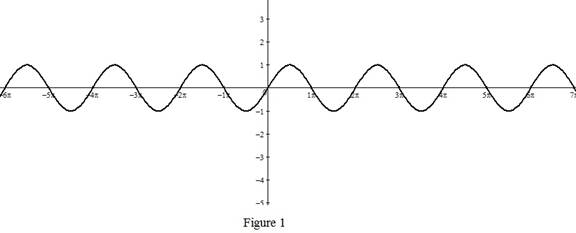From Figure 1, it is observed that the sine function is defined on 1sinx1 and is a periodic function with period 2π,sin(x+2π)=sin(x).

(b)

To determine

Expert Solution

### Explanation of Solution

The rough graph of y=tanx is roughly drawn and shown below in Figure 2.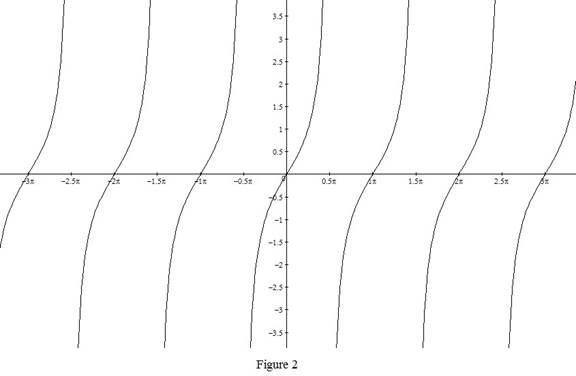From Figure 2, it is observed that the tan function is undefined when cos x = 0 and is a periodic with period π,tan(x+π)=tan(x).

(c)

To determine

Expert Solution

### Explanation of Solution

The rough graph of y=ex is roughly drawn and shown below in Figure 3.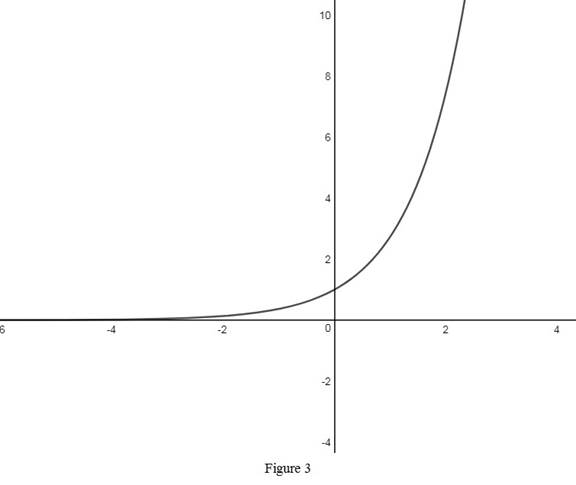From Figure 3, it is observed that the exponential function is an increasing function with the domain (,) and the range (0,).

(d)

To determine

Expert Solution

### Explanation of Solution

The rough graph of y=lnx is roughly drawn and shown below in Figure 4.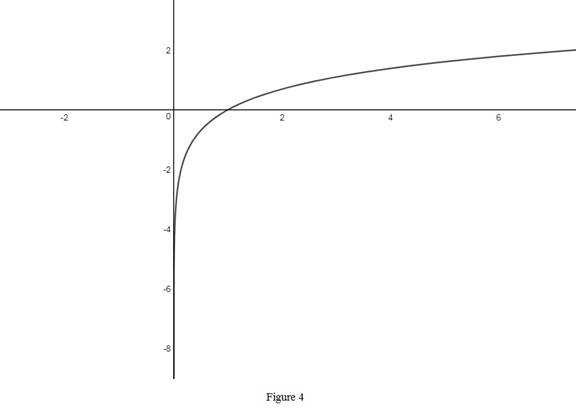From Figure 4, it is observed that the logarithmic function is an increasing function with the domain (0,) and the range (,). Also, notice that the logarithmic function is a reciprocal of an exponential function.

(e)

To determine

Expert Solution

### Explanation of Solution

The rough graph of y=1x is roughly drawn and shown below in Figure 5.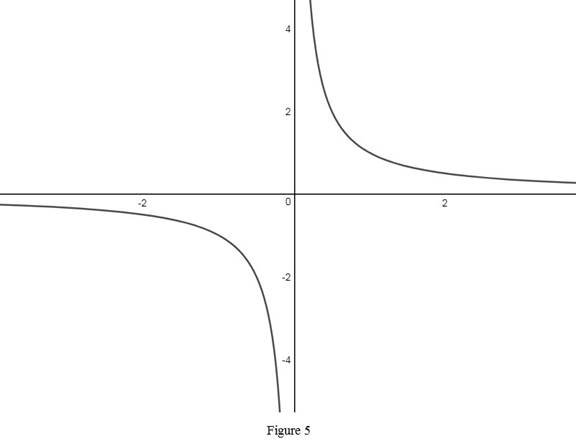From Figure 5, it is observed that the reciprocal function is an hyperbola with x and y axes as its asymptotes.

(f)

To determine

Expert Solution

### Explanation of Solution

The rough graph of y=|x| is roughly drawn and shown below in Figure 6.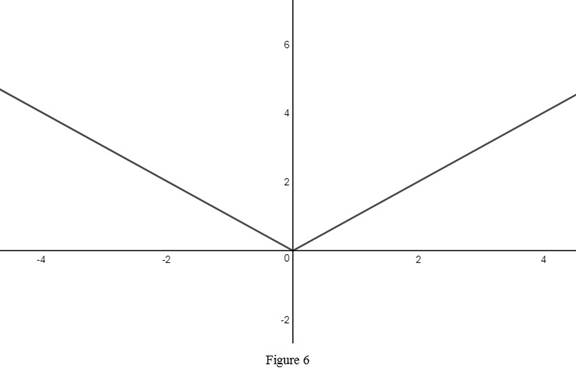From Figure 6, it is observed that the range of an absolute function is positive y-axis.

(g)

To determine

Expert Solution

### Explanation of Solution

The rough graph of y=x is roughly drawn and shown below in Figure 7.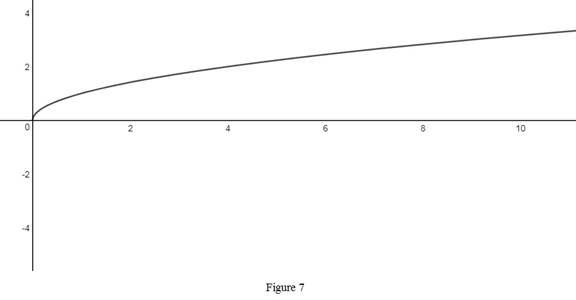From Figure 7, it is observed that the range of the power function with the power 12.

(h)

To determine

Expert Solution

### Explanation of Solution

The rough graph of y=tan1x is roughly drawn and shown below in Figure 8.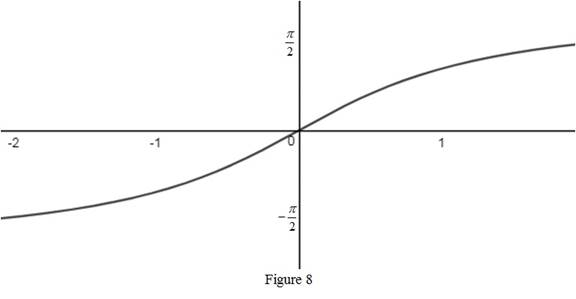From Figure 8, it is observed that the domain of an inverse tan function is (,) and the range is (π2,π2). Also, notice that the tan function and the inverse of trigonometric function are inverse function of each other. So, they reflect about the y = x.

### Have a homework question?

Subscribe to bartleby learn! Ask subject matter experts 30 homework questions each month. Plus, you’ll have access to millions of step-by-step textbook answers!